CAT  >  NMAT Quantitative Skills MCQ Quiz - 4

# NMAT Quantitative Skills MCQ Quiz - 4

Test Description

## 48 Questions MCQ Test NMAT Mock Test Series | NMAT Quantitative Skills MCQ Quiz - 4

NMAT Quantitative Skills MCQ Quiz - 4 for CAT 2022 is part of NMAT Mock Test Series preparation. The NMAT Quantitative Skills MCQ Quiz - 4 questions and answers have been prepared according to the CAT exam syllabus.The NMAT Quantitative Skills MCQ Quiz - 4 MCQs are made for CAT 2022 Exam. Find important definitions, questions, notes, meanings, examples, exercises, MCQs and online tests for NMAT Quantitative Skills MCQ Quiz - 4 below.
Solutions of NMAT Quantitative Skills MCQ Quiz - 4 questions in English are available as part of our NMAT Mock Test Series for CAT & NMAT Quantitative Skills MCQ Quiz - 4 solutions in Hindi for NMAT Mock Test Series course. Download more important topics, notes, lectures and mock test series for CAT Exam by signing up for free. Attempt NMAT Quantitative Skills MCQ Quiz - 4 | 48 questions in 60 minutes | Mock test for CAT preparation | Free important questions MCQ to study NMAT Mock Test Series for CAT Exam | Download free PDF with solutions
 1 Crore+ students have signed up on EduRev. Have you?
NMAT Quantitative Skills MCQ Quiz - 4 - Question 1

### The question is followed by two statements, A and B. Choose the most appropriate option given below. A ladder is inclined from the ground onto the fifth floor of a building. What is the length of the ladder? A. The ladder makes an angle of 56.87° with the ground and the building has fifteen floors in all. B. The bottom of the ladder and the building are 47.89 m apart and the ladder is inclined at an angle of 45° with the ground.

Detailed Solution for NMAT Quantitative Skills MCQ Quiz - 4 - Question 1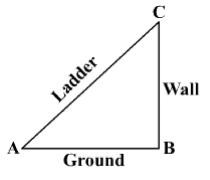Using statement A alone: Only the angle of inclination is known. Since the height of each floor is unknown, the height of the building cannot be found. So, the length of the ladder cannot be found.

Thus, the question cannot be answered using statement A alone.
Using statement B alone: Since the ladder is inclined at 45° with the ground, the angle of elevation is 45°.
Hence, the height of the fifth floor of the building is equal to the distance between the base of the building and ladder.
Thus, the triangle so formed is a right isosceles triangle (with sides equal to 47.89 m) and the length of the ladder is its hypotenuse.
So, the length of the ladder can be found.
Thus, the question can be answered using statement B alone.
Thus, the question can be answered using statement B alone, but cannot be answered using statement A alone.

Hence, option 2.

NMAT Quantitative Skills MCQ Quiz - 4 - Question 2

### The question is followed by two statements, A and B. Choose the most appropriate option given below. What is the value of x and y? x and y are integers. I. 12x - 4y = 48 and 30x -  l0y = 120 II. 32x = 45y

Detailed Solution for NMAT Quantitative Skills MCQ Quiz - 4 - Question 2

Using Statement I alone: 12x - 4y = 48 Multiply the above equation by 2.5 to get: 30x - 10y = 120 Thus for the two equations, we get: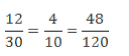Thus, infinite solutions exist for the given equation and a unique value of x and j cannot be found.
Thus, the question cannot be answered using statement I alone.
Using Statement II alone:32x = 45y
This equation is satisfied only for x =y = 0 Thus, the question can be answered using statement II alone.
Thus, the question can be answered using statement II alone but not by using statement I alone. Hence, option 2

NMAT Quantitative Skills MCQ Quiz - 4 - Question 3

### The question is followed by two statements, A and B. Choose the most appropriate option given below. What is the profit percentage made by a shopkeeper if he sells one dozen bananas? I. The cost price of a banana is equal to half the selling price. II. The profit is same as the cost price.

Detailed Solution for NMAT Quantitative Skills MCQ Quiz - 4 - Question 3

Using statement I alone: SP = 2CP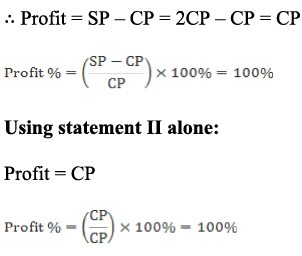Both the statements independently answer the question.
Hence, option 3.

NMAT Quantitative Skills MCQ Quiz - 4 - Question 4

The question is followed by two statements, A and B. Choose the most appropriate option given below.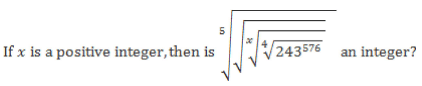A. x is a multiple of 7.
B. x = 4n where n is an integer such that n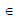[0, 3).

Detailed Solution for NMAT Quantitative Skills MCQ Quiz - 4 - Question 4Thus, statement A alone is sufficient to answer the question.
Using statement B alone: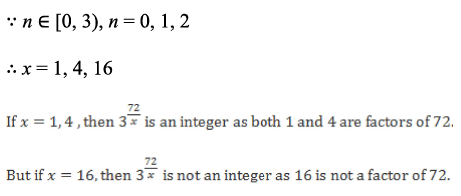Thus, statement B alone is not sufficient to answer the question. Hence, option 1.

NMAT Quantitative Skills MCQ Quiz - 4 - Question 5

The question is followed by two statements, A and B. Choose the most appropriate option given below.

What is the area of triangle ABC?

A. The length of the circumradius is 4 cm.

B. The perimeter of the triangle is 36 cm and the triangle has the maximum possible area.

Detailed Solution for NMAT Quantitative Skills MCQ Quiz - 4 - Question 5

Using Statement A alone:

Area of triangle = abc\4r

where a, b, c are the sides of the triangle and R is the circumradius Since the length of sides is not known, the area cannot be found. Thus, the question cannot be answered using statement A alone.
Using Statement B alone: Since the triangle has the maximum possible area, it has to be an equilateral triangle Hence, each side of the triangle = 36/3 = 12 cm Once the length of each side of a triangle, its semi-perimeter and area can be found.
Thus, the question can be answered using statement B alone.
Thus, the question can be answered using statement B alone but not by using statement A alone.
Hence, option 2.

NMAT Quantitative Skills MCQ Quiz - 4 - Question 6

The question is followed by two statements, A and B. Choose the most appropriate option given below.

What will be the date on the last Saturday of April 2064?

A. 6th April 2064 will be a Friday.

B. The last day of April 2064 will be a Thursday.

Detailed Solution for NMAT Quantitative Skills MCQ Quiz - 4 - Question 6

Using statement A alone: Since 6th April 2064 will be a Friday, 7th, 14th, 21st and 28th April 2064 will be Saturdays.
So, the last Saturday of April 2064 will be on the 28th.

Thus, the question can be answered using statement A alone.
Using statement B alone: Since the last day of April 2064 will be a Thursday, 30th April will be a Thursday.
Thus, 25th April will be the last Saturday of the month.
Thus, the question can be answered using statement B alone.
Thus, the question can be answered using either statement alone.
Hence, option 3.

NMAT Quantitative Skills MCQ Quiz - 4 - Question 7

A and B are employed to complete a work. When A worked at twice his normal efficiency and B worked at half his normal efficiency, the work was completed in 17 days. Had A worked at five times his normal efficiency and B worked at one-fourth of his normal efficiency, the work would have been completed in 10 days. If B works alone, how many days does he need to complete the work?

Detailed Solution for NMAT Quantitative Skills MCQ Quiz - 4 - Question 7

Let the total work be the LCM of 17 and 10 i.e. 170 units.
Let A do a units of work per day.
Since B works at half or one-fourth of his normal efficiency, let B do 4b units of work per day.
In the first case, work is completed in 17 days. 17(2a + 2b) = 170. 2a + 2b= 10.  a + b = 5 ... (i) In the second case, work is completed in 10 days. 10(5a + b) = 170  5a + b = 17 ... (ii)

Solving (i) and (ii), a = 3 and b = 2. Hence, amount of work done by B per day = 4b = 8 units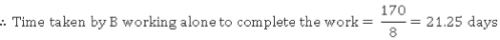Hence, option 1.

NMAT Quantitative Skills MCQ Quiz - 4 - Question 8

What is the number of 4-digit numbers which when divided by 7 and 9 leave a remainder of 3 in each case?

Detailed Solution for NMAT Quantitative Skills MCQ Quiz - 4 - Question 8

Basically we need to find out the count of 4-digit number of the form: [m x L.C.M.(7, 9) + 3] i.e. of the form 63m + 3, where m is a natural number.
The total numbers below 10000 and of the form 63m + 3 are 158 and the largest such number is (63 x 158 + 3) = 9957. Similarly the total numbers below 1000 and of the form 63m + 3 are 15 and the largest such number is (63 x 15 + 3) = 948 Thus the number of 4-digit numbers which when divided by 7 and 9 leave a remainder of 3 in each case are 158 - 15 = 143 Hence, option 3.

NMAT Quantitative Skills MCQ Quiz - 4 - Question 9

There is a cube of volume 125 m3. Its faces are to be covered by sticking handmade paper and wrapping paper on them for a craft project in the following way. Three faces of the cube are to be covered fully with handmade paper. Two other faces are to be covered using 4 strips of wrapping paper and 4 strips of handmade paper for each face, each strip of an area equal to (1/8)th of a face of the cube. The remaining face is to be covered half by each kind of paper. What is the product of the surface area of the cube covered by handmade paper and that covered by wrapping paper?

Detailed Solution for NMAT Quantitative Skills MCQ Quiz - 4 - Question 9

Volume of the cube = 125 m3
Length of each side of the cube = 5 m.  Area of each face of the cube = 5 x 5 = 25 m2

3 faces are covered fully by handmade paper. 2 faces are covered with 4 strips of each paper, each strip being of (1/8)th of the area of the cube.
Therefore, each type of paper covers half of each of the 2 faces. Another face is wrapped half by each paper.

Faces covered with handmade paper = 3 + 0.5 + 0.5 + 0.5 = 4.5. Area covered with handmade paper = 4.5 x 25 - Faces covered with wrapping paper = 6 - 4.5 = 1.5.  Area covered with wrapping paper = 1.5 x 25. Required product of the areas = 4.5 x 25 x 1.5 x 25 = 4218.75 m2
Hence, option 3.

NMAT Quantitative Skills MCQ Quiz - 4 - Question 10

A shopkeeper sells a TV at 20% profit. The cost price of this TV and selling price of a radio differ by Rs. 100, with the cost price of the TV being higher. He incurs a loss of 25% on the transaction of the radio. What is the net profit/loss in the two transactions taken together?

Detailed Solution for NMAT Quantitative Skills MCQ Quiz - 4 - Question 10

Let the CP of the TV be Rs. x; SP of TV = Rs. 1.2x.

Since the cost price of the TV exceeds the selling price of the radio by Rs. 100, SP of radio = Rs. (x - 100). Since the shopkeeper incurs a loss of 25% on the radio transaction, CP of the radio = (x - 100)/0.75 = Rs. 1.33(x - 100).

Total CP = x + 1.33(x - 100) = Rs. (2.33x - 133) and total SP = 1.2x + (x - 100) = Rs. (2.2x - 100). Since the value of x is not known, the net profit/loss cannot be found.
Hence, option 5.

NMAT Quantitative Skills MCQ Quiz - 4 - Question 11

ABCDEF is a regular hexagon. A circle passes through the vertices A and D as shown below. If the radius of the circle is 10 cm, and the centre of the circle is 6 cm away from AD, what is the distance of BC from the centre of the circle?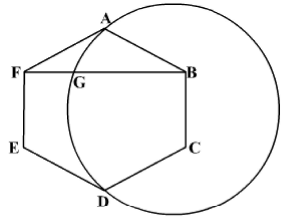Detailed Solution for NMAT Quantitative Skills MCQ Quiz - 4 - Question 11

Let the centre O of the circle meet the chord AD at point P as shown below.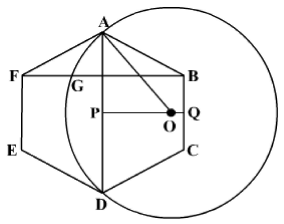Hence, OP = 6 cm and OA = radius of circle = 10 cm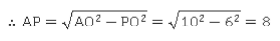Since ABCDEF is a regular hexagon, AP = PD = BC = BP = 8 cm Let O meet BC at Q such that OQ = x cm.
Hence, in ΔPBQ, BP2 = BQ2 + PQ2

Now, BQ = BC/2 = 4 cm and PQ - PO + OQ = (x + 6) cm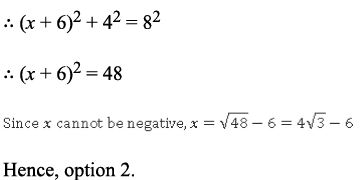NMAT Quantitative Skills MCQ Quiz - 4 - Question 12

There are three groups of students M1, M2 and M3, having 20, 30 and 40 students respectively. If one student each from M1 and M3 leave their respective groups and join M2, the average weight of M1 and M3 decreases by 2 kg each and the average weight of M2 increases by 3 kg. By how much (in kg) does twice the average weight of M2 differ from sum of the average weights of M1 and M3?

Detailed Solution for NMAT Quantitative Skills MCQ Quiz - 4 - Question 12

Let the average weight of the students in M1, M2 and M3 be a, b and c kg respectively.
Since the student leaving M1 has decreased the average weight of the group, this student is heavier than the average weight.
Now, note that if there are x objects and the average value decreases by p, the total value should have decreased by xp.
Since there are 19 students now in M1, and the average weight has reduced by 2 kg, the total weight of the group should have reduced by 2 x 19 = 38 kg.
So, the weight of the student who left M1 would be (a + 38) kg.
Similarly, the student who left M3 would be heavier than the average weight of M3.

Since there are 39 students left in M3 and the average weight of this group reduces by 2 kg, the weight of the student who left M3 = (c + 78) kg.
Both these students joined M2, thereby increasing its average weight by 3 kg.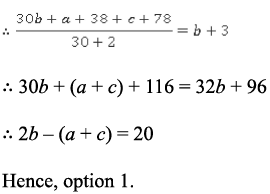NMAT Quantitative Skills MCQ Quiz - 4 - Question 13

Two varieties of cheese i.e. A and B are mixed in the ratio 2:1 and sold at Rs. 46 per kg. If cheese B costs Rs. 50 per kg and the dealer has sold the mixture at cost, what is the price of cheese A (in Rs. per kg)?

Detailed Solution for NMAT Quantitative Skills MCQ Quiz - 4 - Question 13

Since the dealer has sold the mixture at cost, C.R of mixture = S.R of mixture = Rs. 46 per kg Since the cost of cheese B (Rs. 50 per kg) is more than the cost of the mixture, the other cheese should cost less than the mixture.
So, cheese A should cost less than Rs. 46 per kg.
Hence, options 4 and 5 can be eliminated.
Now, the cost of cheese A can be found, as shown below: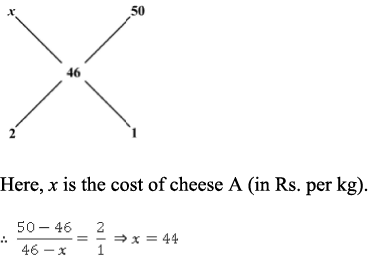Thus, cheese A costs Rs. 44 per kg.
Hence, option 2

NMAT Quantitative Skills MCQ Quiz - 4 - Question 14

The product of digits of a three-digit positive integer n is 6. If n has 16 factors, what is the sum of digits of n?

Detailed Solution for NMAT Quantitative Skills MCQ Quiz - 4 - Question 14

6 = 1 x 2 x 3 = 1 x 1 x 6 So, n can take 9 possible values: 123, 132, 231, 213, 312, 321, 116, 161 and 611.
Now, if the digits are 1, 2 and 3, the sum is 6 while if the digits are 1, 1 and 6, the sum is 8.
Hence, options 1 and 2 can be directly eliminated.
Now, factorise each number.
If a number n is factorised as x its number of factors = (1 + 1)(1 + 1) = (2)(2) = 4.
Hence, if any of the given numbers can be written as a product of exactly two prime factors, it cannot be the value of n (as it will have only 4 factors). 123 = 3 x 41 i.e. rejected.

132 = 22 x 3 x 11 i.e. no. of factors = (2 + 1)(1 + 1)(1 + 1) = 12.

231 = 3 x 7 x 11 i.e. no. of factors = (1 + 1)(1 + 1)(1 + 1) = 8.

213 = 3 x 41 i.e. rejected. 312 = 23 x 3 x13 i.e. no. of factors = (3 + 1)(1 + 1)(1 + 1) = 16. Hence, 312 is a possible value of n.

321 = 3 x 107 i.e. rejected. 116 = 22 x 29 i.e. no. of factors = (2 + 1)(1 + 1) = 6.

161 = 7 x 23 i.e. rejected 611 = 13 x 47 i.e. rejected. Hence, the only possible value of n is 312 and sum of digits of 312 = 3 + 1 + 2 = 6 Hence, option 4.

NMAT Quantitative Skills MCQ Quiz - 4 - Question 15

There are two circular tables with six and eight chairs respectively. In how many ways can 14 people sit around the two circular tables?

Detailed Solution for NMAT Quantitative Skills MCQ Quiz - 4 - Question 15

Let us divide 14 people into group of 6 and 8 people respectively.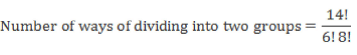Within the two groups, number of ways to arrange 6 and 8 around the table 5! and 7! respectively.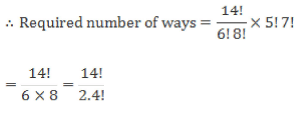NMAT Quantitative Skills MCQ Quiz - 4 - Question 16

On a busy Monday morning a certain number of cyclists started from Borivali towards Churchgate. At the Malad junction, half of these cyclists entered Malad while 1/5 of the remaining cyclists joined the cyclists going towards Chuchgate. The same thing happened at Andheri, Santacruz, Bandra and Dadar. No cyclist joined or left the group thereafter. Finally 486 cyclists reached Churchgate. How many cyclists started from Borivali?

Detailed Solution for NMAT Quantitative Skills MCQ Quiz - 4 - Question 16

There are 486 cyclists at Churchgate. So 486 cyclists left Dadar. Let there be x cyclists before Dadar.
Since half of the cyclists left, there were x/2 cyclists remaining. These were joined by 1/5 of remaining cyclists.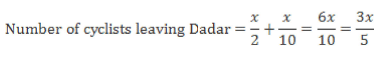This has to be equal to 486. x = 810
Using the same logic, we have the number of cyclists before Bandra to be 1350, before Santacruz to be 2250, before Andheri to be 3750 and before Malad to be 6250.
Thus total cyclists leaving Borivali is 6250.

Hence, option 1.

NMAT Quantitative Skills MCQ Quiz - 4 - Question 17

If A(-7, 5), B(-3, -4) and C(1, k) form an isosceles triangle, k can be,

Detailed Solution for NMAT Quantitative Skills MCQ Quiz - 4 - Question 17

Case 1, if AB = BC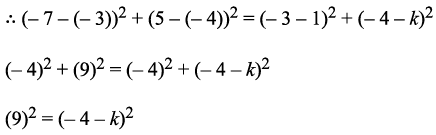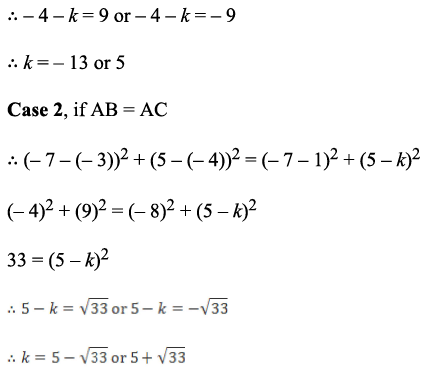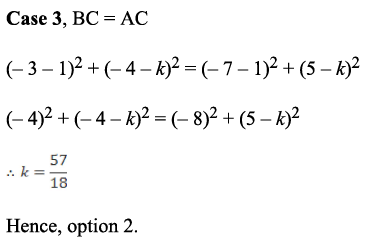NMAT Quantitative Skills MCQ Quiz - 4 - Question 18

Each of Shyam and Ram has some chocolates. If Shyam gives five chocolates to Ram, both will have equal number of chocolates. If Ram gives the same number of chocolates to Shyam, Shyam would have twice the number of chocolates as Ram. How many chocolates does Ram originally have?

Detailed Solution for NMAT Quantitative Skills MCQ Quiz - 4 - Question 18

Let Shyam and Ram originally have s and r chocolates respectively.
When Shyam gives 5 chocolates to Ram; s - 5 = r + 5.

s - r = 10 ... (i) When Ram gives 5 chocolates to Shyam; s + 5 = 2(r - 5). So 2r - s = 15 ... (ii) Adding (i) and (ii); r = 25 Hence, option 4.

NMAT Quantitative Skills MCQ Quiz - 4 - Question 19

nth term of an AP, consisting of only positive integers, is denoted by an. It is given that a= 4 and aN - 2 = 9. What is the value of (aN + 2N + 2)?

Detailed Solution for NMAT Quantitative Skills MCQ Quiz - 4 - Question 19

Let common difference of the AP be d.
We have a1 = a3 - 2d = 4 - 2d.

We also have a1 = aN - 2 - ( N - 3 )d = 9 - d( N - 3) = 9 - N d + 3d Equating these two values of a1, we get,

4 - 2d = 9 -Nd + 3d, or (N - 5)d = 5.

Since both N and d are positive, we must have N - 5 = 1 and d = 5 or N - 5 = 5 and d = 1.
Thus, either N = 6 and d = 5 or N = 10 and d = 1.
In the first case, since d = 5, a1 = 4 - 2d = 4-2*5 = -6.
But it is given in the question that the terms are all positive integers. So this case is not possible.
In the second case, aN + 2N + 2 = a1+ (N - 1)d + 2N + 2 = 4 - 2 x 1 + 9 x 1 + 2 x 10 + 2 = 33.

So value of the given expression = 33 Hence, option 3.

NMAT Quantitative Skills MCQ Quiz - 4 - Question 20

What is the radius of the largest circle drawn between the lines 3x + 4y - 5 = 0 and 6x + 8y - 15 = 0?

Detailed Solution for NMAT Quantitative Skills MCQ Quiz - 4 - Question 20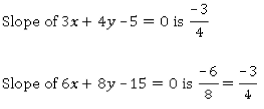Since the two lines have the same slope, they are parallel to each other. Hence, the largest circle drawn between these lines touches both these lines. Hence, distance between these lines = diameter of the circle For two parallel lines with equations ax + by + c1 = 0 and ax + by + C2 = 0,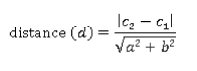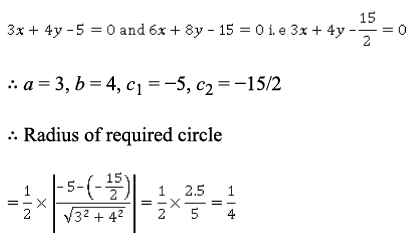Hence, option 2.

NMAT Quantitative Skills MCQ Quiz - 4 - Question 21

Aladdin tosses five coins. What is the probability of getting even number of heads and odd number of tails?

Detailed Solution for NMAT Quantitative Skills MCQ Quiz - 4 - Question 21

When a coin is tossed, there are two equally likely outcomes possible, head and tail.
Hence, number o f possible outcomes when five coins are tossed = 2 x 2 x 2 x 2 x 2 = 25 = 32. Even number of heads and odd number of tails gives three cases: 0 heads and 5 tails. This is like arranging a five-letter word where all the letters are same. This can be done in only 1 way. 2 heads and 3 tails. This is like arranging a five-letter word where one letter is present twice and the other is present thrice.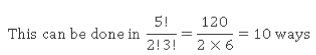4 heads and 1 tail.

This is again like arranging a five-letter word where one letter is present four times and the other is present just once.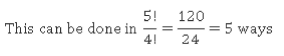Hence, total ways of getting even number of heads and odd number of tails = 10 + 5 + 1 = 16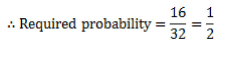Hence, option 1.

NMAT Quantitative Skills MCQ Quiz - 4 - Question 22

For a function defined as m(x) = 4x3 - 5x2 + 2x + 13, it is known that m(0) < k + 5 and m(1) > k - 15. Which of the following can be a value of k?

Detailed Solution for NMAT Quantitative Skills MCQ Quiz - 4 - Question 22

m( 0) = 4(0)3 - 5(0)2 + 2(0) +13 = 13.

m(0) < k + 5. 13 < k + 5. k > 8

Hence, options 1 and 4 can be eliminated. m(1) = 4(1)3 - 5(1)2 + 2(1) + 13 = 4 - 5 + 2 + 13 = 14.

Since m(1) > k - 15

14 > k - 15
Therefore k < 29 i.e. 8 < k< 29. Among the options, only k = 10 satisfies this condition
Hence, option 3.

NMAT Quantitative Skills MCQ Quiz - 4 - Question 23

The average amount with x students in a class is Rs. 35. If 4 students with an average amount of Rs.15 leave the group, the average amount now w ith the students would be the same as the amount if 2 students with an average amount of Rs. 90 join the group. How many students does the class have?

Detailed Solution for NMAT Quantitative Skills MCQ Quiz - 4 - Question 23

The total amount with the students in the class is Rs. 35x. If 4 students with an average amount of Rs.15 leave the group, the average amount now left would be the same as the amount if 2 students with an average amount of Rs. 90 join the group.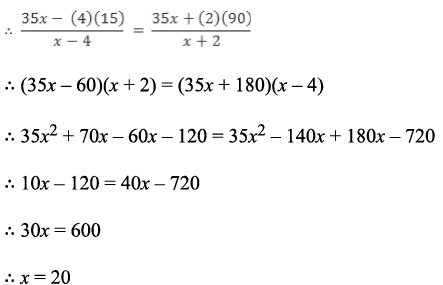Thus, the class has 20 students.
Hence, option 3.

NMAT Quantitative Skills MCQ Quiz - 4 - Question 24

There are 2 metal pillars k m apart such that the height of one metal pillar is twice that of the other. Standing exactly between the base of the two pillars, a tourist observes that the angle of elevation to the top of the two pillars forms a pair of complementary angles. What is the height (in m) of the shorter pillar?

Detailed Solution for NMAT Quantitative Skills MCQ Quiz - 4 - Question 24

The given data can be represented as shown below: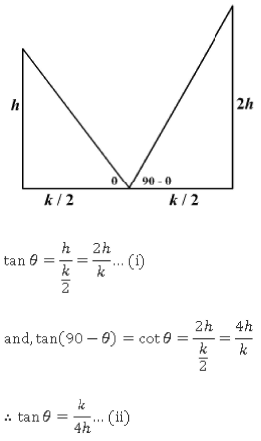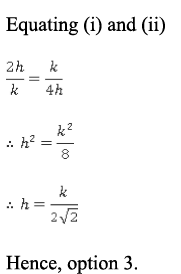NMAT Quantitative Skills MCQ Quiz - 4 - Question 25

How many real values of x satisfy the equation.

log(x + 1)(2x - 1) + 2 log(2x - 1)(x + 1) = 3?

Detailed Solution for NMAT Quantitative Skills MCQ Quiz - 4 - Question 25

The given equation can be written as: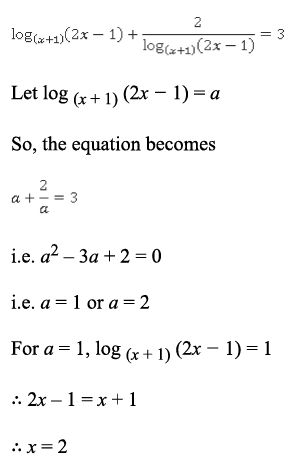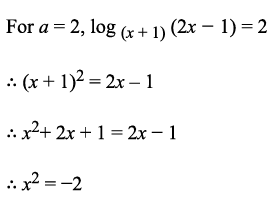Since x is real, this case is not possible.
Thus, only one real value of x satisfies the given equation.
Hence, option 1.

NMAT Quantitative Skills MCQ Quiz - 4 - Question 26

A clock is set at 6 a.m. Let the time when the minute and hour hand are together for the first time and the second time be x and y respectively. What is y - x?

Detailed Solution for NMAT Quantitative Skills MCQ Quiz - 4 - Question 26

When the hour hand and minute hand are together, the angle between the two hands is 0°.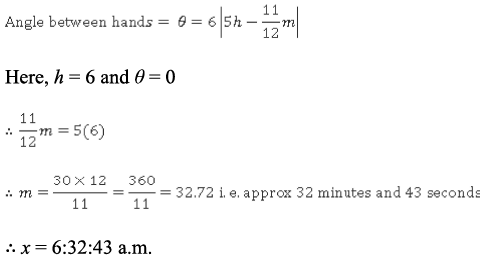The hands meet again between 7 a.m. and 8 a.m.
Here, h = 7 and θ = 0.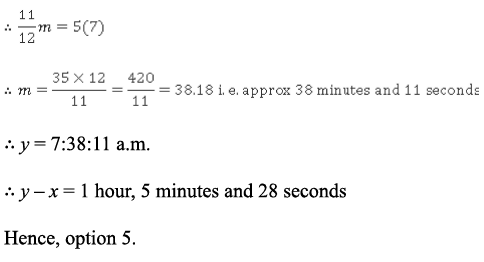NMAT Quantitative Skills MCQ Quiz - 4 - Question 27

After selling 70% of the articles on 40% discount shopkeeper announces another 30% discount on the remaining articles. After selling all the articles he makes net profit of 20%. Then find the actual cost price as a ratio of their marked price if the cost price of all articles is equal and so is their marked price?

Detailed Solution for NMAT Quantitative Skills MCQ Quiz - 4 - Question 27

Without losing generality we can assume that shopkeeper has 100 articles with marked price of 100.
Now, for first 70 articles he offered discount of Rs. 40 on each of the articles.
Hence selling price of these 70 articles will be Rs. 60 each. Total selling price of these 70 articles will be, 70 x 60 = 4200 And he offers additional 30% discount on remaining articles.
Hence selling price of these 30 articles will be 70% of 60 i.e. Rs. 42 each. Total selling price of these 30 articles will be, 30 x 42 = 1260. Total selling price of 100 articles is, 1260 + 4200 = 5460.
This is 20% more than cost price. Hence,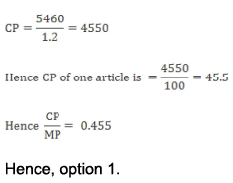NMAT Quantitative Skills MCQ Quiz - 4 - Question 28

How many integral values of x satisfy |x + 1| + |x| + |x - 1| + |x - 2| = 7?

Detailed Solution for NMAT Quantitative Skills MCQ Quiz - 4 - Question 28

Note that ( x - 2), ( x - 1), x and (x + 1) are four consecutive integers.
The magnitude of no set of four consecutive integers adds up to 7.
Hence, no integral values of x satisfy the given equation. Hence, option 1.

NMAT Quantitative Skills MCQ Quiz - 4 - Question 29

Group Question

Answer the following question based on the information given below.

The table below shows the percentage breakup of the fields in which the students that passed from a B-school applied for jobs.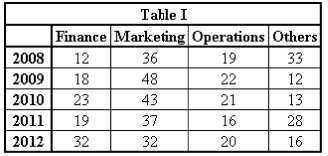The table below shows the average starting salary for some of these fields.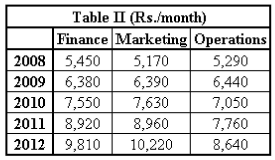The graph below shows the number of students that passed out from this B-school in each year. Only students who passed out could apply for jobs, and all of them did so.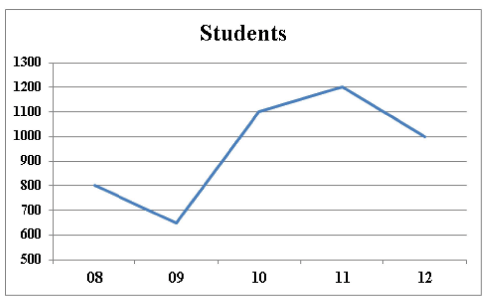Q. If ‘Others’ comprises only Systems and HR, and the number of students applying for HR jobs each year was the same, what is the least number of students who applied for Systems jobs over the given period?

Detailed Solution for NMAT Quantitative Skills MCQ Quiz - 4 - Question 29

Only students who passed could apply for jobs and all such students applied for jobs.
Number of students applying for a job in ‘Others’ = (0.33 x 800) + (0.12 x 650) + (0.13 x 1100) + (0.28 x 1200) + (0.16 x 1000) = 264 + 78 + 143 + 336+ 160
= 981

Since the number of students applying for Systems jobs has to be mimimized, the number of students applying for HR should be the highest possible.
The number of students applying for HR jobs was the same each year.
Hence, this number is maximized when there are 78 applicants for HR each year (as number of students each year is equal and there cannot be more than 78 applicants in 2009).
Maximum number of students applying for an HR job = 78 x 5 = 390 Minimum number of students applying for a Systems job = 981 - 390 = 591
Hence, option 2.

NMAT Quantitative Skills MCQ Quiz - 4 - Question 30

The table below shows the percentage breakup of the fields in which the students that passed from a B-school applied for jobs.The table below shows the average starting salary for some of these fields.The graph below shows the number of students that passed out from this B-school in each year. Only students who passed out could apply for jobs, and all of them did so.Q. In 2009, 25% of the students applying for Marketing jobs applied to Nefertiti, a confectionary major. If only half of them were selected and Nefertiti paid them 10% above the average starting salary, what was Nefertiti’s monthly salary outflow on these recruits?

Detailed Solution for NMAT Quantitative Skills MCQ Quiz - 4 - Question 30

In 2009, students applying to Nefertiti = 25% of 48% of 650 Also, students selected in Nefertiti = 50% of 25% of 48% of 650 = 0.5 x 0.25 x 0.48 x 650
= 39
Each got a salary that was 10% above the starting salary paid in Marketing.
Total monthly salary outflow for Nefertiti = 39 x 1.1 x 6390 = Rs. 2,74131.
Hence, option 3.

NMAT Quantitative Skills MCQ Quiz - 4 - Question 31

The table below shows the percentage breakup of the fields in which the students that passed from a B-school applied for jobs.The table below shows the average starting salary for some of these fields.The graph below shows the number of students that passed out from this B-school in each year. Only students who passed out could apply for jobs, and all of them did so.Q. By what percentage did the average salary for this B-school increase from 2010 to 2011?

Detailed Solution for NMAT Quantitative Skills MCQ Quiz - 4 - Question 31

Since the average salary for Others is not known, the average salary for the B- school cannot be found for any year.
Hence, option 5.

NMAT Quantitative Skills MCQ Quiz - 4 - Question 32

The table below shows the percentage breakup of the fields in which the students that passed from a B-school applied for jobs.The table below shows the average starting salary for some of these fields.The graph below shows the number of students that passed out from this B-school in each year. Only students who passed out could apply for jobs, and all of them did so.Q. The total amount earned by the students applying for Finance jobs in 2010 was what percent of the total amount earned by the students appyling for Operations jobs in 2012?

Detailed Solution for NMAT Quantitative Skills MCQ Quiz - 4 - Question 32

The total amount earned by students applying for Finance jobs in 2010  = 0.23 * 1100 x 7550 = Rs. 19,10,150.

The total amount earned by students applying for Operations jobs in 2012 = 0.2 x 1000 x 8640 = Rs. 17,28,000 Thus, the required percentage is definitely greater than 100.
Hence, options 1 and 3 can be directly eliminated.
Required percentage = (1910150/1728000) x 100 ⇒ 110% Hence, option 4.

NMAT Quantitative Skills MCQ Quiz - 4 - Question 33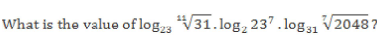Detailed Solution for NMAT Quantitative Skills MCQ Quiz - 4 - Question 33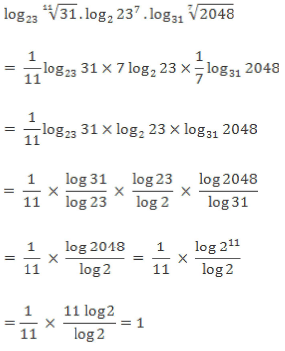Hence, option 4.

NMAT Quantitative Skills MCQ Quiz - 4 - Question 34

A wooden box contains exactly 12 nuts and 24 bolts. One-third of the bolts and one-third of the nuts are defective. If two items are picked from the box up one after the other without replacement, what is the probability that neither item is defective?

Detailed Solution for NMAT Quantitative Skills MCQ Quiz - 4 - Question 34

Out of 12 nuts, 4 are defective while out of 24 bolts, 8 are defective.
So, there are 12 defective items and 24 non-defective items.
So, the probability that both items are non-defective (when picked without replacement one after the other)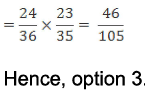NMAT Quantitative Skills MCQ Quiz - 4 - Question 35

MN is a chord of a circle of radius 14 cm. Chord MN subtends a right angle at the center O of the circle. Find the area of the minor segment MCNO, where C is a point on minor arc MN.

Detailed Solution for NMAT Quantitative Skills MCQ Quiz - 4 - Question 35

A(minor segment MCNO) = A(minor sector MCNO) - A(ΔMON)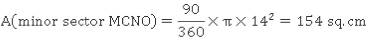Since MN subtends a right angle at the centre of the circle, MNO is an isosceles right triangle with base = height = 14 cm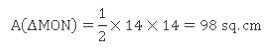A(minor segment MCNO) = 154 - 98 = 56 sq.cm Hence, option 2.

NMAT Quantitative Skills MCQ Quiz - 4 - Question 36

Which of the following statements is/are true?

a. A perfect square always has an odd numbers of factors.

b. If a number n has five factors between 1 and the square root of n (n is a perfect square), then n has 13 factors.

c. 72 has 11 factors.

Detailed Solution for NMAT Quantitative Skills MCQ Quiz - 4 - Question 36

A perfect square is of the form n2a Number of factors = (2a + 1), which is odd Hence, statement (a) is correct.
Hence, options 2 and 5 can be eliminated.
Since statement (c) is easier to check using a direct formula, check for statement (c) first.

72 = 23 x 32

Number of factors = (3 + 1)(2 + 1) = 12 Thus, statement (c) is incorrect.
Hence, options 3 and 4 can be eliminated.
Hence, option 1.
Note: Statement (a) can also be checked by considering 2 or 3 random perfect squares and finding their number of factors.
Statement (b) can be verified as under:

N is a perfect square and has 5 factors between 1 and √n.
Half of the factors of a number which is a perfect square are lesser than its square root and half of them are greater than its square root. e.g., 36 has the factors 1, 2, 3, 4, 6, 9, 12, 18 and 36 and √36= 6 1,2, 3 and 4 are less than 6 while 9, 12, 18 and 36 are greater than 6. If the number of factors of n between 1 and √n = 5, then the number of factors between √n and n is also 5.

Apart from this, 1, √n and n are also factors of n.
Total number of factors = 5 + 5 + 3=13.

NMAT Quantitative Skills MCQ Quiz - 4 - Question 37

Group Question

Answer the following question based on the information given below.

In a certain country, the movement of the oil and gas industry is dependent on the movement of 3 stocks, A, B and C.
The charts below show the performance of the three stocks over a period of 10 days. X can correspond to any of three stocks, and so can Y.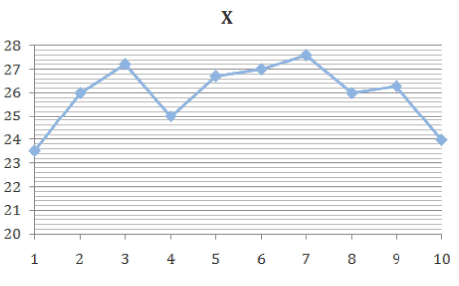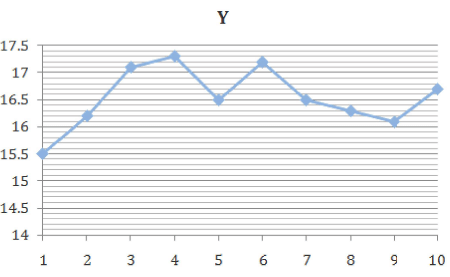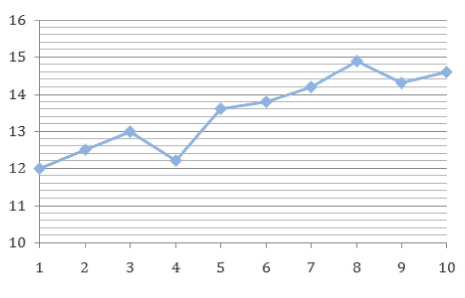The oil and gas index of the country is calculated as 3A + 2B + C. The index is said to have ‘Risen’ if all the stocks go up on that day. It is said to be ‘steady’ if 2 stocks go up that day. It is said to be “declining” if only a single stock goes up while it is said to have “plunged” if none of the stocks go up that day.

Answer the following questions based on the information above.

Q. How many days did the index “decline”?

Detailed Solution for NMAT Quantitative Skills MCQ Quiz - 4 - Question 37

For the index to ‘decline’, only one stock should go up on that particular day.
This is satisfied only on days 4, 8 and 9.
Hence, the index ‘declined’ on three days.
Hence, option 3.

NMAT Quantitative Skills MCQ Quiz - 4 - Question 38

In a certain country, the movement of the oil and gas industry is dependent on the movement of 3 stocks, A, B and C.
The charts below show the performance of the three stocks over a period of 10 days. X can correspond to any of three stocks, and so can Y.The oil and gas index of the country is calculated as 3A + 2B + C. The index is said to have ‘Risen’ if all the stocks go up on that day. It is said to be ‘steady’ if 2 stocks go up that day. It is said to be “declining” if only a single stock goes up while it is said to have “plunged” if none of the stocks go up that day.

Answer the following questions based on the information above.

Q. What is the maximum rise in the value of index from day 1 to day 10?

Detailed Solution for NMAT Quantitative Skills MCQ Quiz - 4 - Question 38

Value of index = 3A + 2B + C

The maximum rise in index would occur when the index has its least possible value on day 1 and highest possible value on day 10.
In the index above, A is multiplied by the largest number (i.e. 3) and B is multiplied by the second largest number (i.e 2).
Hence, to get the minimum value on day 1, take A = 12, B = 15.5 and C = 23.5.

Index on day 1 = (3 * 12) + (2 * 15.5) + 23.5 = 36 + 31 + 23.5 = 90.5 Similarly, index on day 10 = (3 * 14.6) + (2 * 16.7) + 24 = 43.8 + 33.4 + 24 = 101.2
Rise in index = 101.2 - 90.5 = 10.7 Required percentage = (10.7/90.5) x 100 = 11.82%

Hence, option 3.

NMAT Quantitative Skills MCQ Quiz - 4 - Question 39

In a certain country, the movement of the oil and gas industry is dependent on the movement of 3 stocks, A, B and C.
The charts below show the performance of the three stocks over a period of 10 days. X can correspond to any of three stocks, and so can Y.The oil and gas index of the country is calculated as 3A + 2B + C. The index is said to have ‘Risen’ if all the stocks go up on that day. It is said to be ‘steady’ if 2 stocks go up that day. It is said to be “declining” if only a single stock goes up while it is said to have “plunged” if none of the stocks go up that day.

Answer the following questions based on the information above.

Q. What is the least possible value of the index?

Detailed Solution for NMAT Quantitative Skills MCQ Quiz - 4 - Question 39

Consider the solution to the previous question.
Observe that each graph has its least possible value on day 1.
Since the index = 3A + 2B + C, the least value of the index is when A = 12, B = 1.5 and C = 23.5 Hence, as calculate earlier, least value of the index = 90.5 Hence, option 5.

NMAT Quantitative Skills MCQ Quiz - 4 - Question 40

In a certain country, the movement of the oil and gas industry is dependent on the movement of 3 stocks, A, B and C.
The charts below show the performance of the three stocks over a period of 10 days. X can correspond to any of three stocks, and so can Y.The oil and gas index of the country is calculated as 3A + 2B + C. The index is said to have ‘Risen’ if all the stocks go up on that day. It is said to be ‘steady’ if 2 stocks go up that day. It is said to be “declining” if only a single stock goes up while it is said to have “plunged” if none of the stocks go up that day.

Answer the following questions based on the information above.

Q. If the value of the index is 105.5 on day 1, what is its value on day 3?

Detailed Solution for NMAT Quantitative Skills MCQ Quiz - 4 - Question 40

Index = 3A + 2B + C
The value of the index on day 1 is 105.5 Because there are three graphs, there are 3! = 6 possibilities.

1) X = C, Y = B, Graph 3 = A => Here, index = 90.5 (as calculated earlier)

2) X = C, Y = A, Graph 3 = B => Here, index = 3(15.5) + 2(12) + 23.5 = 46.5 + 24 + 23.5 = 94
3) X = B, Y = C, Graph 3 = A => Here, index = 3(12) + 2(23.5) + 15.5 = 36 + 47 + 15.5 = 98.5
4) X = B, Y = A, Graph 3 = C => Here, index = 3(15.5) + 2(23.5) + 12 = 46.5 + 47 + 12 = 105.5
Since the correct value of the index has been obtained, you do not need to calculate the remaining two casese.

Using these values, index on day 3 = 3(17.1) + 2(27.2) + 13 = 51.3 + 54.4 + 13 = 118.8. Hence, option 1.

NMAT Quantitative Skills MCQ Quiz - 4 - Question 41

On Monday, Mohsin increased the speed of car by 25% as compared to his usual speed and reached office 16 minutes earlier. On Tuesday, Mohsin took a longer route to the office and travelled with his usual speed. If on Tuesday, he reached office 20 minutes late, then how many percent more did Mohsin travel to reach office?

Detailed Solution for NMAT Quantitative Skills MCQ Quiz - 4 - Question 41

Let the usual speed of Mohsin be x.
Mohsin’s speed on Monday = 1.25x Assume that usually Mohsin takes t minutes to reach the office.
Time taken on M onday = t - 16. Distance travelled = xt =1.25x (t - 16) Solving this, we get

f = 80 minutes
On Tuesday, Mohsin reached office 20 minutes late, travelling with his usual speed, i.e., he travelled for (20/80 =) 25% more.
As the speed with which he travelled was same, distance a time.
As time taken is 25% more, the distance travelled will also be 25% more.
Hence, option 3.

NMAT Quantitative Skills MCQ Quiz - 4 - Question 42

A parallelogram with an internal angle of 150° has an area of 48 sq.cm and a perimeter of 40 cm. What is the length of the adjacent sides of the parallelogram (in cm)?

Detailed Solution for NMAT Quantitative Skills MCQ Quiz - 4 - Question 42

Since one internal angle of the parallelogram is 150°, the other has to be 30°. Let the adjacent sides of the parallelogram measure a cm and b cm respectively.
Perimeter = 2 (a + b) = 40 i.e. a + b = 20 ... (i) Area = ab * sin 30 = 48. ab = 48 x 2 = 96 ... (ii) Dividing (i) by (ii)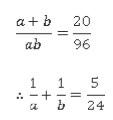The only values of a and b (from the options) that satisfy this equation are 12 and 8. Hence, option 1.

NMAT Quantitative Skills MCQ Quiz - 4 - Question 43

What is the range of x that satisfies the inequality |3x2 - 5| > 5x + 3

Detailed Solution for NMAT Quantitative Skills MCQ Quiz - 4 - Question 43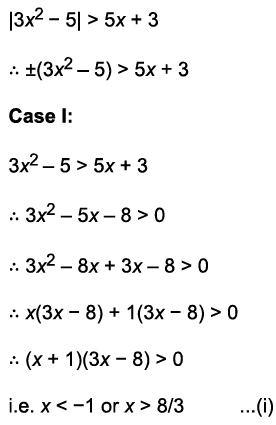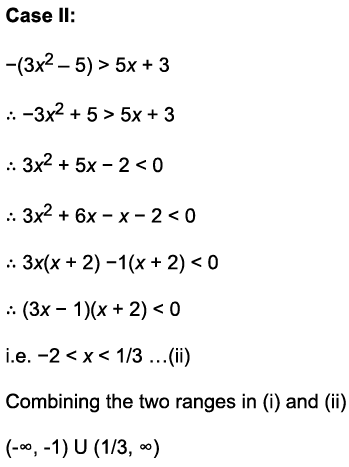Hence, option 5.

NMAT Quantitative Skills MCQ Quiz - 4 - Question 44

Group Question

Answer the following question based on the information given below.

The table below gives the national income and the population of a country for the years 2009-10 to 2014-15.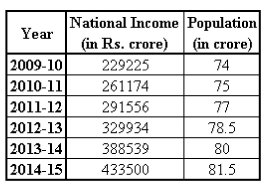Q. What was the average of the annual per capita income (in Rs.) over the given  period?

Detailed Solution for NMAT Quantitative Skills MCQ Quiz - 4 - Question 44

Per capita income = total income / total population Since the question asks for the average of the annual per capita income, first find the per capita income for each year (as shown below).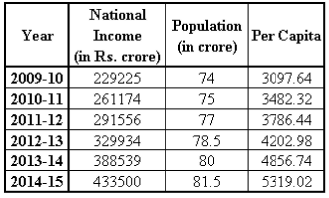Average of this per capita income (in Rs.) = 4124.19 Hence, option 2.

NMAT Quantitative Skills MCQ Quiz - 4 - Question 45

The table below gives the national income and the population of a country for the years 2009-10 to 2014-15.Q. What is the difference (in Rs. crore) between the average national income for the given period and the national income for the year having the highest per capita income?

Detailed Solution for NMAT Quantitative Skills MCQ Quiz - 4 - Question 45

Consider the solution to the first question.
From the table, the highest per capita income is in 2014-15.
The national income for this year is Rs. 4,33,500 crore.
Average national income = Rs. 322321.33 crore. Required difference = 433500 - 322321.33 = 111178.67 Hence, option 4.

NMAT Quantitative Skills MCQ Quiz - 4 - Question 46

The table below gives the national income and the population of a country for the years 2009-10 to 2014-15.Q. Which year sees the least percentage growth in the per capita income?

Detailed Solution for NMAT Quantitative Skills MCQ Quiz - 4 - Question 46

Consider the solution to the first question.
First calculate the absolute change in the per capita income for each and see if some options can be eliminated.

2010- 11: Change = 3482.32 - 3097.64 = 384.68 and base = 3097.64 i.e. % increase > 10%

2011- 12: 3786.44 - 3482.32 = 304.12 and base = 3482.32 i.e. % increase < 10% 2012- 13: 4202.98 - 3786.44 = 416.54 and base = 3786.44 i.e. % increase > 10% 2013- 14: 4856.74 - 4202.98 = 653.76 and base = 4202.98 i.e. % increase > 10% 2014- 15: 5319.02 - 4856.74 = 462.28 and base = 4856.74 i.e. increase < 10% Hence, the least percentage growth is in 2011-12 or 2014-15 % growth in 2011-12 = (304.12/3482.32) x 100 = 8.73% % growth in 2014-15 = (462.28/4856.74) x 100 = 9.52%

Hence, option 4.

NMAT Quantitative Skills MCQ Quiz - 4 - Question 47

The table below gives the national income and the population of a country for the years 2009-10 to 2014-15.Q. In 2015-16, the national income and per capita income increased by 25% and 35% respectively. If the population of each year was to be ranked from  onwards in ascending order of population, where would 2015-16 be ranked?

Detailed Solution for NMAT Quantitative Skills MCQ Quiz - 4 - Question 47

Consider the solutions to the previous questions.
National income in 2015-16 = 433500 * 1.25 = 541875 Per capita income in 2015-16 = 5319.02 x 1.35 = 7180.68 Population in 2015-16 = 541875/7180.68 = 75.46 Hence, in ascending order of population, 2015-16 would be ranked third.
Hence, option 5

NMAT Quantitative Skills MCQ Quiz - 4 - Question 48

A alone can complete some work in 6 days more than what B alone needs to complete it. A started the work and worked alone for 7 days. As soon as he left, B took over and completed the work in 10 more days. In how many days can A and B individually complete the work?

Detailed Solution for NMAT Quantitative Skills MCQ Quiz - 4 - Question 48

Option 3 can be directly eliminated as B can finish the complete work in 9 days in that case, whereas B takes 10 days to complete partial work.
Let B and A take x and x + 6 days to complete the work individually.
Hence,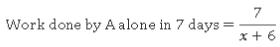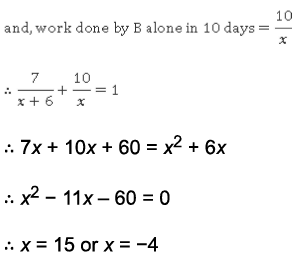Since number of days cannot be negative, x = 15 Thus, B and A can do the work in 15 and 15 + 6 = 21 days respectively.
Hence, option 1.

## NMAT Mock Test Series

1 docs|23 tests
 Use Code STAYHOME200 and get INR 200 additional OFF Use Coupon Code
Information about NMAT Quantitative Skills MCQ Quiz - 4 Page
In this test you can find the Exam questions for NMAT Quantitative Skills MCQ Quiz - 4 solved & explained in the simplest way possible. Besides giving Questions and answers for NMAT Quantitative Skills MCQ Quiz - 4, EduRev gives you an ample number of Online tests for practice

## NMAT Mock Test Series

1 docs|23 tests

### How to Prepare for CAT

Read our guide to prepare for CAT which is created by Toppers & the best Teachers